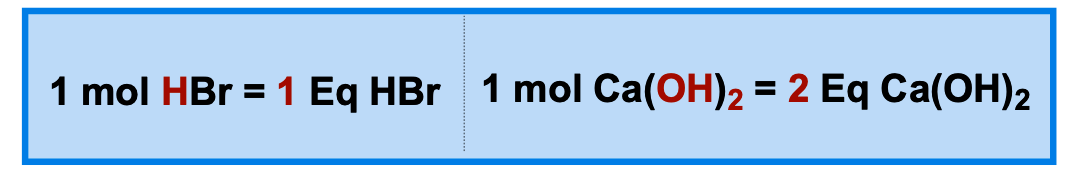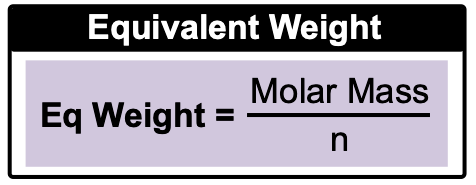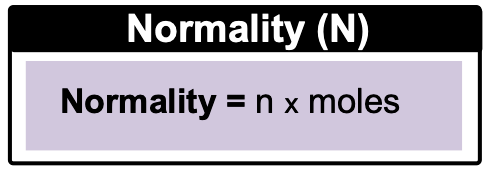Clutch Prep is now a part of Pearson
Ch.10 Acids and BasesWorksheetSee all chapters

# Acid-Base Equivalents

See all sections
Sections
Acid-Base Introduction
Arrhenius Acid and Base
Bronsted Lowry Acid and Base
Acid and Base Strength
Ka and Kb
The pH Scale
Auto-Ionization
pH of Strong Acids & Bases
Acid-Base Equivalents
Acid-Base Reactions
Gas Evolution Equations (Simplified)
Ionic Salts (Simplified)
Buffers
Henderson-Hasselbalch Equation
Strong Acid Strong Base Titrations (Simplified)

Acid-Base Equivalents are used to measure the number of H+ ions or OH- ions in acids and bases, respectively.

###### Understanding Acid-Base Equivalents

Concept #1: Understanding Acid-Base EquivalentsConcept #2: Calculating Acid-Base Equivalents

Example #1: Calculate number of Equivalents in each of the following:

a) 1 mole of H3PO4                     b) 2.7 g of RbOH

Concept #3: Equivalent WeightExample #2: Calculate the equivalent weight of H2SO4.

Concept #4: NormalityExample #3: Calculate Normality of each of the following solutions:

a) 4.6 x 10-2 M NaOH                             b) 0.35 g of H3POin 1 L

Practice: Calculate mass (grams) needed for the following base equivalent: 0.18 mEq of Mg(OH)2.

Practice: Identify the acid that possesses an equivalent weight of 63 grams.

Practice: Determine volume (mL) needed to prepare a 0.73 g of Ca(OH)2 solution with 1.25 N.